+关注继续查看

# 线性分类

• 分类器必须记忆所有的训练数据，储存起来，以便和未来的测试数据进行比较。这个对空间效率性很不友好，因为数据集很容易达到G级别的大小；
• 对测试图像进行分类代价很大，因为它需要和整个的训练集图片进行比较；

# 线性分数函数（从图像到标签分数的参数化映射）

f:RDRK

## 线性分类器。

f(xi,W,b)=Wxi+b

• 首先，注意这个单独的矩阵Wxi可以有效地对10组不同的分类数据同时进行处理，每一个类代表W向量中的一行；
• 同样注意，在这里我们认为输入数据(xi,yi)是特定的、经过修正的，但是我们可以对参数W,b进行任意修改。我们的目的是将这些参数进行设定，从而可以实现计算出来的分数匹配整个训练集中的真实数据。我们将会对这个内容进行深入分析，但是直观上我们希望正确分类的分数比其他错误分类的分数更高。
• 这种方法的一个优点是，训练数据用来学习参数W,b，但是一旦学习完成我们可以扔掉整个训练集而只需要保存参数。这是因为一个新的测试图像只需要进入进入这个方程中，被分类，得到计算后的分数即可。
• 最后注意，对测试图像进行分类中涉及到了一个矩阵乘法和矩阵加法，这比将测试数据和整个训练数据进行比较可快多了。

# 线性分类器的解释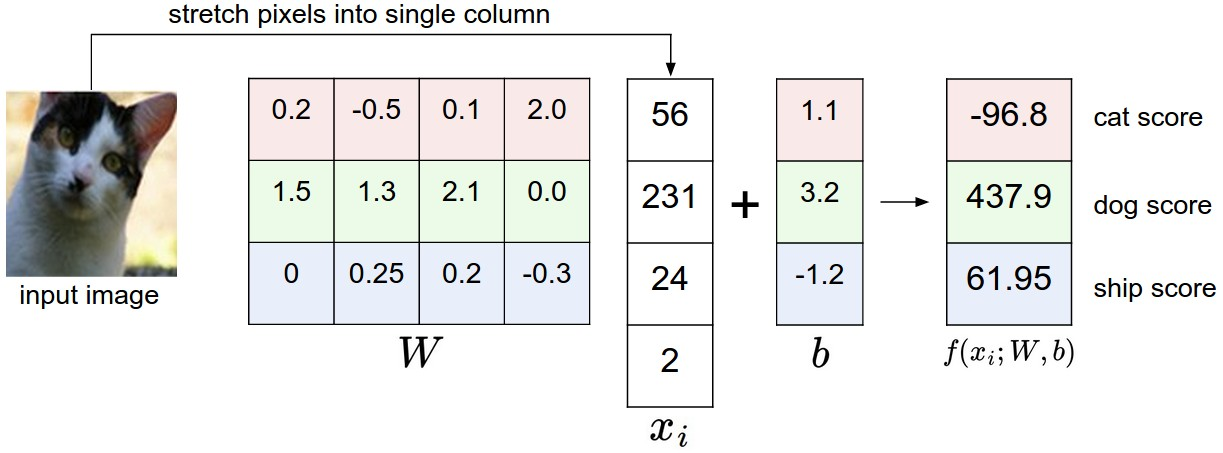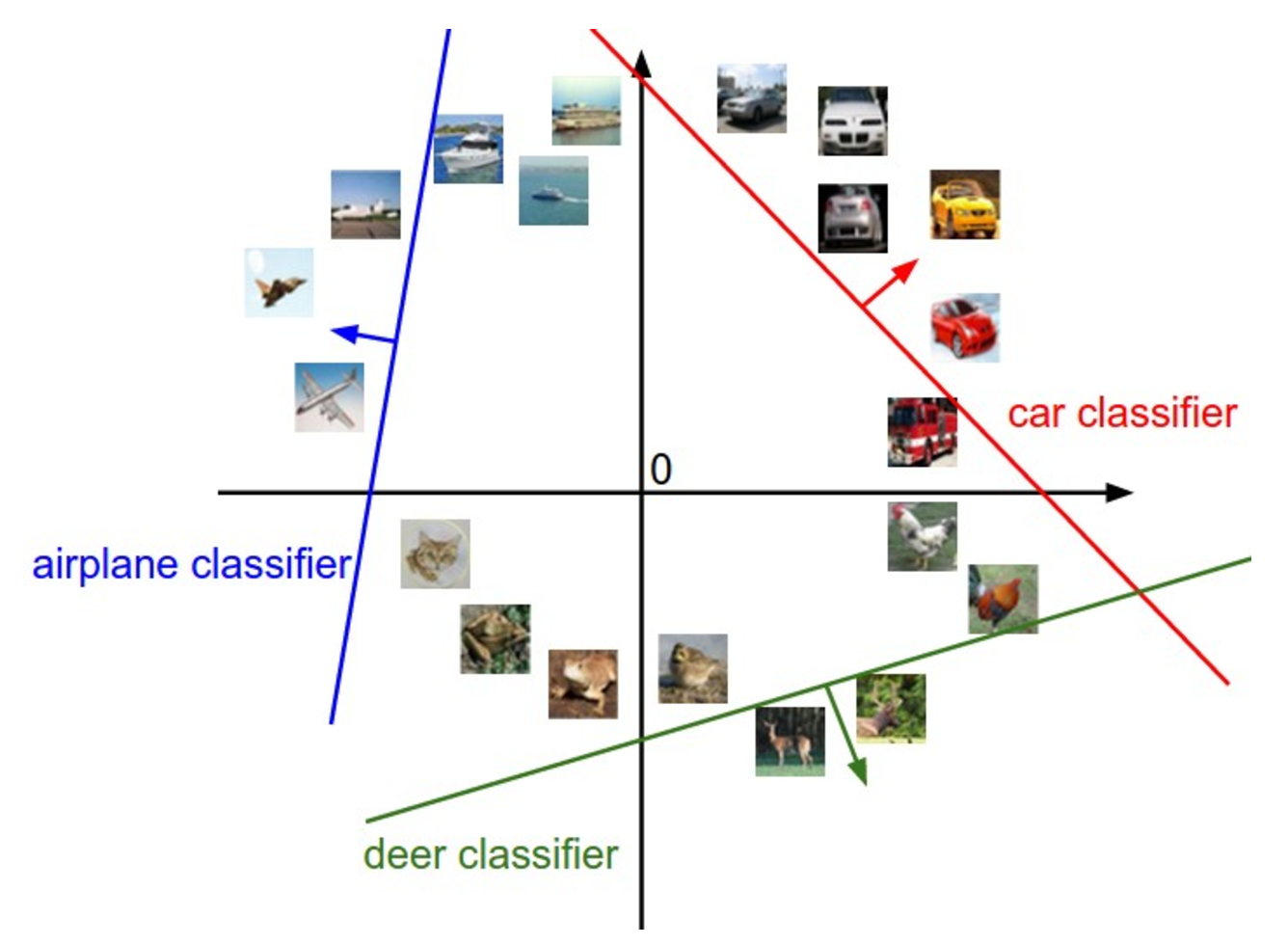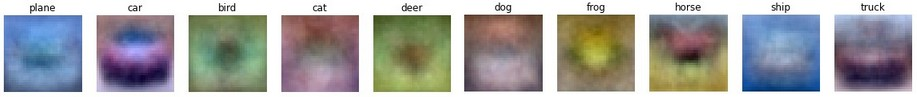f(xi,W,b)=Wxi+b

f(xi,W)=Wxi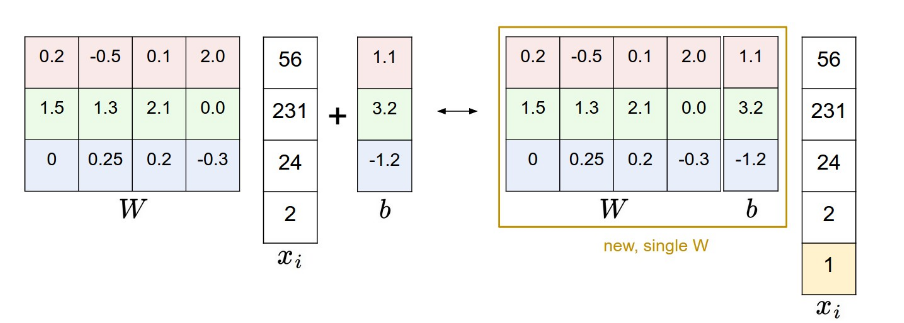# 损失函数

## 多类支持向量机损失

Li=jyimax(0,sjsyi+Δ)

Li=max(0,713+10)+max(0,1113+10)

Li=jyimax(0,wTjxiwTyixi+Δ)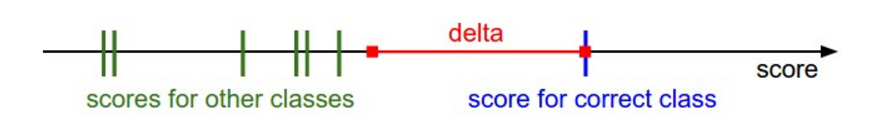R(W)=klW2k,l

L=1NiLidata loss+λR(W)regularization loss

L=1Nijyi[max(0,f(xi;W)jf(xi;W)yi+Δ)]+λklW2k,l

def L_i(x, y, W):
"""
无向量版本，对一个简单的例子（x，y）进行多分类svm损失计算
- x是一个表示图像的列向量(e.g. 3073 x 1 in CIFAR-10)，在3073列包含一个偏移向量(i.e. bias trick)
- y是一个给与了正确分类索引的整数(e.g. between 0 and 9 in CIFAR-10)
- w是权重矩阵(e.g. 10 x 3073 in CIFAR-10)
"""
delta = 1.0 # 在下节中再讲解这个值
scores = W.dot(x) # 得到的scores的形式为10 x 1, 也就是十个类别
correct_class_score = scores[y]
D = W.shape #分类数量, e.g. 10
loss_i = 0.0
for j in xrange(D): # 对所有错的分类进行迭代
if j == y:
# 省略正确的分类，仅对不正确的分类进行迭代
continue
# 累计第i个分类的损失
loss_i += max(0, scores[j] - correct_class_score + delta)
return loss_i

def L_i_vectorized(x, y, W):
"""
一个快速的半向量优化，半向量指的是对一个简单的例子来说，对循环进行了优化，但是依然存在一个循环（在这个函数外头）
"""
delta = 1.0
scores = W.dot(x)
# 在一个向量操作中计算所有的分类的矩阵
margins = np.maximum(0, scores - scores[y] + delta)
# 在第y个位置 scores[y] - scores[y] 取消了，多了delta。我们想要忽略第y个位置，仅仅考虑在最大错的分类中的差值
margins[y] = 0
loss_i = np.sum(margins)
return loss_i

def L(X, y, W):
"""
标准向量优化 :
- X holds all the training examples as columns (e.g. 3073 x 50,000 in CIFAR-10)
- y is array of integers specifying correct class (e.g. 50,000-D array)
- W are weights (e.g. 10 x 3073)
"""
# evaluate loss over all examples in X without using any for loops
# left as exercise to reader in the assignment

https://zhuanlan.zhihu.com/p/20900216?refer=intelligentunit

|
2月前
|

66 0
|
2月前
|

【深度学习】实验16 使用CNN完成MNIST手写体识别(PyTorch)
【深度学习】实验16 使用CNN完成MNIST手写体识别(PyTorch)
47 0
|
2月前
|

【深度学习】实验15 使用CNN完成MNIST手写体识别(Keras)
【深度学习】实验15 使用CNN完成MNIST手写体识别(Keras)
26 0
|
2月前
|

【深度学习】实验14 使用CNN完成MNIST手写体识别(TensorFlow)
【深度学习】实验14 使用CNN完成MNIST手写体识别(TensorFlow)
39 0
|
3月前
|

55 0
|
5月前
|

122 0
|
6月前
|

【深度学习】基于卷积神经网络的天气识别训练
【深度学习】基于卷积神经网络的天气识别训练
116 0
|
7月前
|

61 0
|
7月前
|

144 0